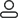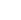LanguageLogin|Sign up
Blog >> Blog Details Page
Analyze Characteristic Impedance--Basic Concept
Posted:03:23 PM July 16, 2018 writer: G

What is a transmission line?

Two conductors of a certain length constitute the transmission line. One of the conductors becomes the channel for signal propagation, and the other conductor constitutes the return path of the signal. Here we refer to the return path of the signal, which is actually what we usually understand, but for the convenience of the description, forget about it for the time being. This concept.). In a multi-layer circuit board design, each PCB interconnect constitutes one conductor in the transmission line, which uses the adjacent reference plane as the second conductor of the transmission line or the return path of the signal. What kind of PCB interconnection is a good transmission line? Usually, if the characteristic impedance is consistent at the same PCB interconnection, such a transmission line becomes a high-quality transmission line. What kind of board is called a controlled impedance board? The circuit board with controlled impedance means that the characteristic impedance of all transmission lines on the PCB meets the uniform target specification, which usually means that the characteristic impedance of all transmission lines is between 25Ω and 70Ω.

From the perspective of signal

The most effective way to consider the characteristic impedance is to look at what the signal itself sees as the signal propagates along the transmission line. To simplify the discussion of the problem, it is assumed that the transmission line is of the microstrip type and the cross-sections of the transmission lines are consistent as the signals propagate along the transmission line.

A step signal having an amplitude of 1 V is added to the transmission line. The step signal is a 1V battery that is connected by the front end and connected between the signal line and the return path. At the instant of turning on the battery, the signal voltage waveform will travel in the dielectric at the speed of light, typically at a speed of about 6 inches/ns (why the signal travels so fast, rather than approaching the speed of electron propagation by about 1 cm/s, which is another topic, do not introduce further here). Of course, here the signal still has a conventional definition, the signal is defined as the voltage difference between the signal line and the return path, always obtained by measuring the voltage difference between the signal return path of any point on the transmission line.

The signal is forwarded at a speed of 6 inches/ns along the direction of the transmission line. What happens to the signal during the transmission? During the first 10 ps interval, the signal traveled a distance of 0.06 inches along the transmission line. Assuming that the lock time is at this moment, consider the situation in which the transmission line occurs. At this distance of travel, the transmission of the signal establishes a constant signal of a constant amplitude of 1V between the transmission line and the corresponding adjacent signal return channel. This means that an additional positive charge and an additional negative charge have accumulated on this segment of the transmission line and the corresponding return path to establishing this stable voltage. It is also the difference in these charges that establishes and maintains a stable 1 V voltage signal between the two conductors, and a stable voltage signal between the conductors creates a capacitance between the two conductors.

It is not clear that there is a signal to propagate over the transmission line at this point in time. Therefore, the voltage between the signal line and the return path is still zero. During the next 10 ps interval, the signal travels a certain distance along the transmission line. The result of the signal continuing to propagate is another 1V between the transmission line segment of 0.06-inch length and the corresponding signal return path. Signal voltage. In order to do this, a certain amount of positive charge must be injected into the signal line while an equal amount of negative charge is injected into the return path of the signal. Each time the signal propagates along the transmission line for a length of 0.06 inches, a more positive charge is injected into the signal line, and more negative charge is injected into the signal return path. At every 10 ps interval, another transmission line is charged to 1 V, and the signal continues to travel forward along the transmission line.

Where do these charges come from? The answer comes from the source, which is the battery we use to provide the step signal and connect to the front of the transmission line. As the signal propagates on the transmission line, the signal continuously charges the transmitted transmission line segment, ensuring that a voltage of 1 V is established and maintained between the signal line and the return path where the signal is transmitted. At every 10 ps interval, the signal propagates a certain distance on the transmission line and draws a certain amount of charge δQ from the power system. The battery provides a constant amount of charge δQ outwardly over a period of time δt, resulting in a constant signal current. Positive current flows from the battery into the signal line, while a negative current of the same magnitude flows through the return path of the signal.

The negative current flowing through the signal return path is exactly the same as the positive current flowing into the signal line. Moreover, at the position of the signal wavefront, the AC current flows through the capacitance formed by the signal line and the signal return path, completing the signal loop.

607 1 0 1
• PCB
Prototype
• PCB
Assembly
• SMD
Stencil
 Dimensions: (mm) × Quantity: (pcs) 5 5 10 15 20 25 30 40 50 75 100 120 150 200 250 300 350 400 450 500 600 700 800 900 1000 1500 2000 2500 3000 3500 4000 4500 5000 5500 6000 6500 7000 7500 8000 9000 10000 Other Quantities:(quantity*length*width is greater than 10㎡) OK Layers: 1 2 4 6 8 10 12 Thickness: 0.6 mm 0.8 mm 1.0 mm 1.2 mm 1.6 mm 2.0 mm 2.5 mmQuote now